# PSEB 4th Class Maths Solutions Chapter 6 Time Ex 6.1

Punjab State Board PSEB 4th Class Maths Book Solutions Chapter 6 Time Ex 6.1 Textbook Exercise Questions and Answers.

## PSEB Solutions for Class 4 Maths Chapter 6 Time Ex 6.1

Question 1.
Write the time shown in each clock given below:
(a)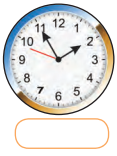Solution:
1:55

(b)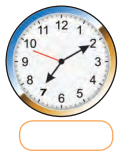Solution:
7:10(c)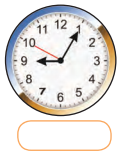Solution:
9:05

(d)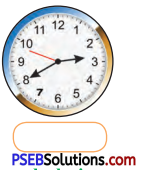Solution:
2:40

(e)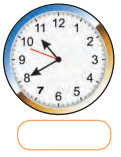Solution:
10:40

(f)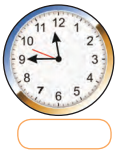Solution:
11:45

Question 2.
Draw clocks in your note book and show the time as given below :
(a) 4:20
(b) 7:35
(c) 4:45
(d) 3:15
(e) 11:40
(f) 9:15.
Solution: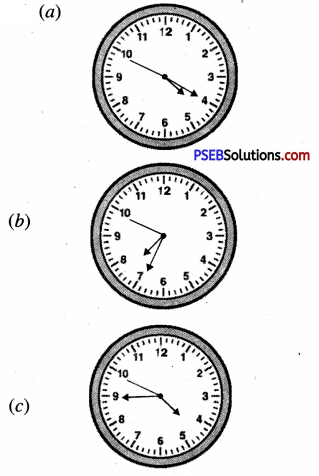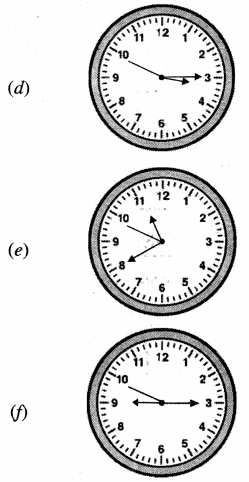Question 3.
How many minutes a minute hand will take to reach the time shown between the first clock and the second clock ?
(a)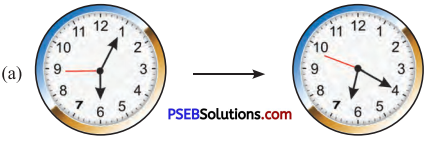Solution:
15 minutes

(b)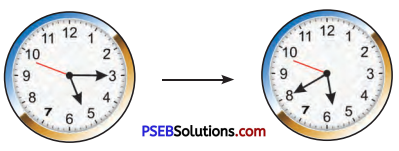Solution:
25 minutes

Question 4.
Tell the time shown in the given clock and write.Solution:
4:18

Question 5.
Tell the time shown in the given clock and write.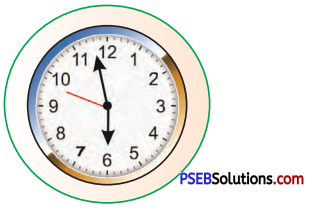Solution:
5:58